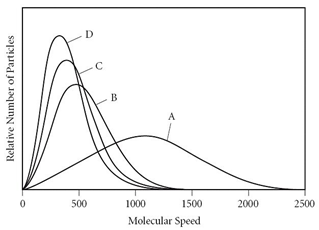# Problem: At constant temperature, which of the following statement(s) is/are  TRUE according to the figure?             I)  Every molecule of gas A has a faster speed than every molecule of gas D. II) Gas D has the smallest molar mass. III) Gas B has a smaller molar mass than gas C.   A) I only       B) II only      C) III only        D) both I and III          E) both II and III

93% (94 ratings)
###### Problem Details

At constant temperature, which of the following statement(s) is/are  TRUE according to the figure?

I)  Every molecule of gas A has a faster speed than every molecule of gas D.

II) Gas D has the smallest molar mass.

III) Gas B has a smaller molar mass than gas C.

A) I only

B) II only

C) III only

D) both I and III

E) both II and IIIFrequently Asked Questions

What scientific concept do you need to know in order to solve this problem?

Our tutors have indicated that to solve this problem you will need to apply the Velocity Distributions concept. You can view video lessons to learn Velocity Distributions. Or if you need more Velocity Distributions practice, you can also practice Velocity Distributions practice problems.

What is the difficulty of this problem?

Our tutors rated the difficulty ofAt constant temperature, which of the following statement(s)...as medium difficulty.

How long does this problem take to solve?

Our expert Chemistry tutor, Sabrina took 2 minutes and 42 seconds to solve this problem. You can follow their steps in the video explanation above.

What professor is this problem relevant for?

Based on our data, we think this problem is relevant for Professor Jursich's class at UIC.